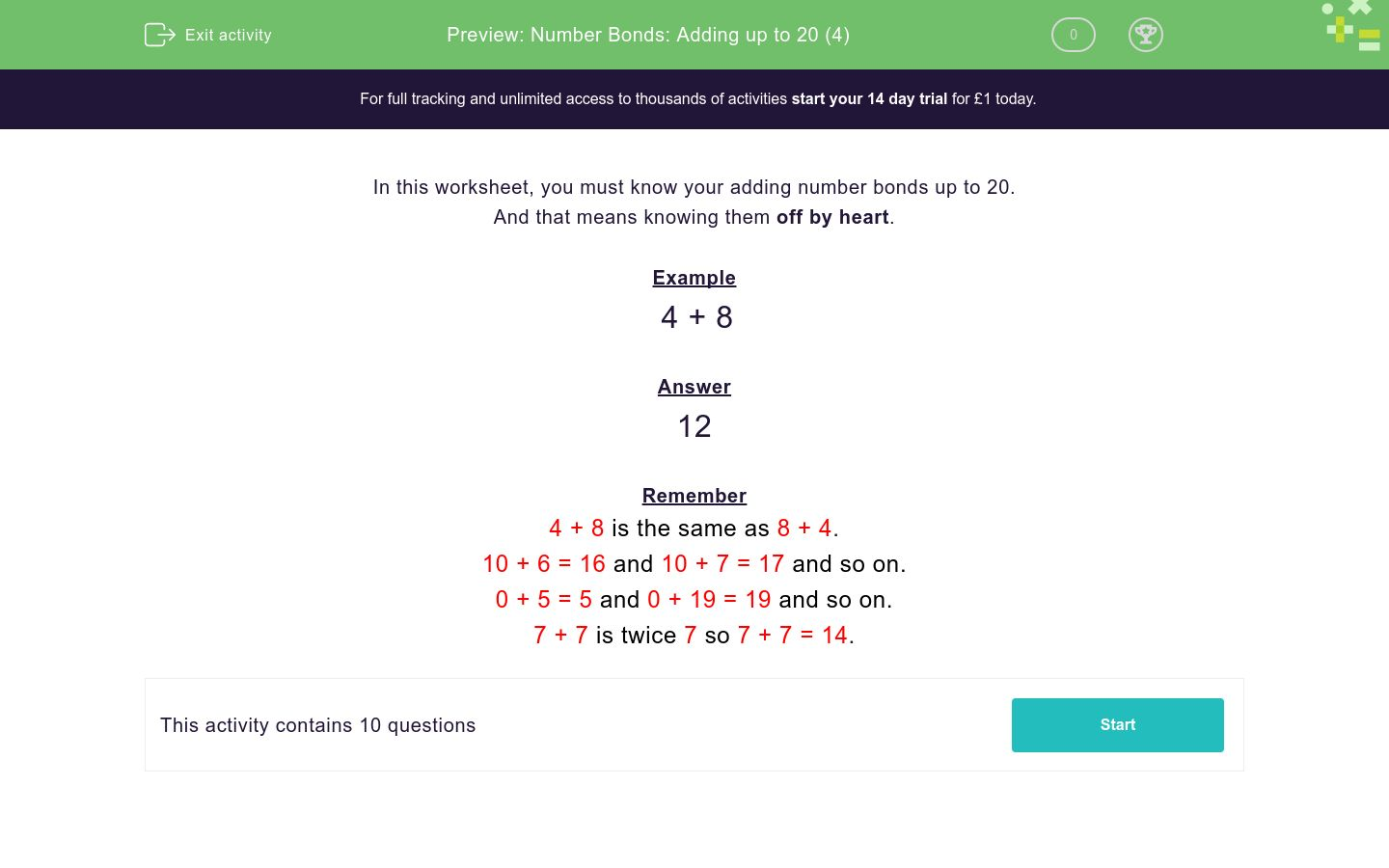# Number Bonds: Adding up to 20 (4)

In this worksheet, students must simply know their addition number bonds up to 20. No explanations are given.Key stage:  KS 1

Curriculum topic:   Number: Addition and Subtraction

Curriculum subtopic:   Add and Subtract to 20

Difficulty level:### QUESTION 1 of 10

In this worksheet, you must know your adding number bonds up to 20.

And that means knowing them off by heart.

Example

4 + 8

12

Remember

4 + 8 is the same as 8 + 4.

10 + 6 = 16 and 10 + 7 = 17 and so on.

0 + 5 = 5 and 0 + 19 = 19 and so on.

7 + 7 is twice 7 so 7 + 7 = 14.

---- OR ----